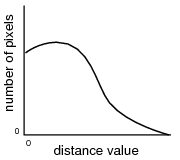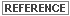# Evaluating Classification

## Producer Field Guide

HGD_Product
Producer Field Guide
HGD_Portfolio_Suite
Producer

After a classification is performed, these methods are available to test the accuracy of the classification:

• Thresholding—Use a probability image file to screen out misclassified pixels.
• Accuracy Assessment—Compare the classification to ground truth or other data.

Thresholding

Thresholding is the process of identifying the pixels in a classified image that are the most likely to be classified incorrectly. These pixels are put into another class (usually class 0). These pixels are identified statistically, based upon the distance measures that were used in the classification decision rule.

Distance File

When a Minimum Distance, Mahalanobis Distance, Maximum Likelihood, Spectral Angle Mapper, or Spectral Correlation Mapper classification is performed, a distance image file can be produced in addition to the output thematic raster layer. A distance image file is a one-band, 32-bit continuous raster layer in which each data file value represents the result of a spectral distance equation, depending upon the decision rule used.

• In a Minimum Distance classification, each distance value is the Euclidean spectral distance between the measurement vector of the pixel and the mean vector of the pixel’s class.
• In a Mahalanobis Distance or Maximum Likelihood classification, the distance value is the Mahalanobis Distance between the measurement vector of the pixel and the mean vector of the pixel’s class.
• In a Spectral Angle Mapper or Spectral Correlation Mapper classification, the distance values are mapped as the sum of the angles of the two spectral curves.

Brighter pixels (containing the higher distance file values) are spectrally farther from the signature means for the classes to which they are assigned. They are more likely to be misclassified.

Darker pixels are spectrally nearer, and more likely to be classified correctly. If supervised training was used, the darkest pixels are usually the training samples.

Histogram of a Distance ImageThe figure above shows how the histogram of the distance image usually appears. This distribution is called a chi-square distribution, as opposed to a normal distribution, which is a symmetrical bell curve.

Threshold

Pixels that are most likely to be misclassified have higher distance file values at the tail of this histogram. At some point that you define—either mathematically or visually—where to cut off the tail of this histogram. The cutoff point is the threshold.

To determine the threshold:

• interactively change the threshold with the mouse, when a distance histogram is displayed while using the threshold function. Use this option to select a chi-square value by selecting the cut-off value in the distance histogram, or
• enter a chi-square parameter or distance measurement, so that the threshold can be calculated statistically.

In both cases, thresholding has the effect of cutting the tail off of the histogram of the distance image file, representing the pixels with the highest distance values.

Chi-square Statistics

If Minimum Distance classifier is used, then the threshold is simply a certain spectral distance. However, if Mahalanobis or Maximum Likelihood are used, then chi-square statistics are used to compare probabilities (Swain and Davis, 1978).

When statistics are used to calculate the threshold, the threshold is more clearly defined as follows:

T is the distance value at which C% of the pixels in a class have a distance value greater than or equal to T.

Where:

T = threshold for a class

C% = percentage of pixels that are believed to be misclassified, known as the confidence level

T is related to the distance values by means of chi-square statistics. The value X2 (chi-squared) is used in the equation. X2 is a function of:

• number of bands of data used—known in chi-square statistics as the number of degrees of freedom
• confidence level

When classifying an image in ERDAS IMAGINE, the classified image automatically has the degrees of freedom (that is, number of bands) used for the classification. The chi-square table is built into the threshold application.

In this application of chi-square statistics, the value of X2 is an approximation. Chi-square statistics are generally applied to independent variables (having no covariance), which is not usually true of image data.

Use Threshold dialog to perform the thresholding.A further discussion of chi-square statistics can be found in a statistics text.

Accuracy Assessment

Accuracy assessment is a general term for comparing the classification to geographical data that are assumed to be true, in order to determine the accuracy of the classification process. Usually, the assumed-true data are derived from ground truth data.

It is usually not practical to ground truth or otherwise test every pixel of a classified image. Therefore, a set of reference pixels is usually used. Reference pixels are points on the classified image for which actual data are (or will be) known. The reference pixels are randomly selected (Congalton, R. 1991).

You can use ERDAS IMAGINE Accuracy Assessment dialog to perform an accuracy assessment for any thematic layer. This layer does not have to be classified by ERDAS IMAGINE (for example, you can run an accuracy assessment on a thematic layer that was classified in a previous version of Hexagon Geospatial software and imported into ERDAS IMAGINE).

Random Reference Pixels

When reference pixels are selected by the analyst, it is often tempting to select the same pixels for testing the classification that were used in the training samples. This biases the test, since the training samples are the basis of the classification. By allowing the reference pixels to be selected at random, the possibility of bias is lessened or eliminated (Congalton, R. 1991).

The number of reference pixels is an important factor in determining the accuracy of the classification. It has been shown that more than 250 reference pixels are needed to estimate the mean accuracy of a class to within plus or minus five percent (Congalton, R. 1991).

ERDAS IMAGINE uses a square window to select the reference pixels. The size of the window can be defined by you. Three different types of distribution are offered for selecting the random pixels:

• random—no rules are used
• stratified random—the number of points is stratified to the distribution of thematic layer classes
• equalized random—each class has an equal number of random points

Use Accuracy Assessment dialog to generate random reference points.

Accuracy Assessment CellArray

An Accuracy Assessment CellArray is created to compare the classified image with reference data. This CellArray is simply a list of class values for the pixels in the classified image file and the class values for the corresponding reference pixels. The class values for the reference pixels are input by you. The CellArray data reside in an image file.

Use Accuracy Assessment CellArray to enter reference pixels for the class values.

Error Reports

From Accuracy Assessment CellArray, two kinds of reports can be derived.

• Error matrix simply compares the reference points to the classified points in a c × c matrix, where c is the number of classes (including class 0).
• Accuracy report calculates statistics of the percentages of accuracy, based upon the results of the error matrix.

When interpreting the reports, it is important to observe the percentage of correctly classified pixels and to determine the nature of errors of the producer and yourself.

Use Accuracy Assessment dialog to generate the error matrix and accuracy reports.

Kappa Coefficient

Kappa coefficient expresses the proportionate reduction in error generated by a classification process compared with the error of a completely random classification. For example, a value of 0.82 implies that the classification process is avoiding 82 percent of the errors that a completely random classification generates (Congalton, R. 1991).